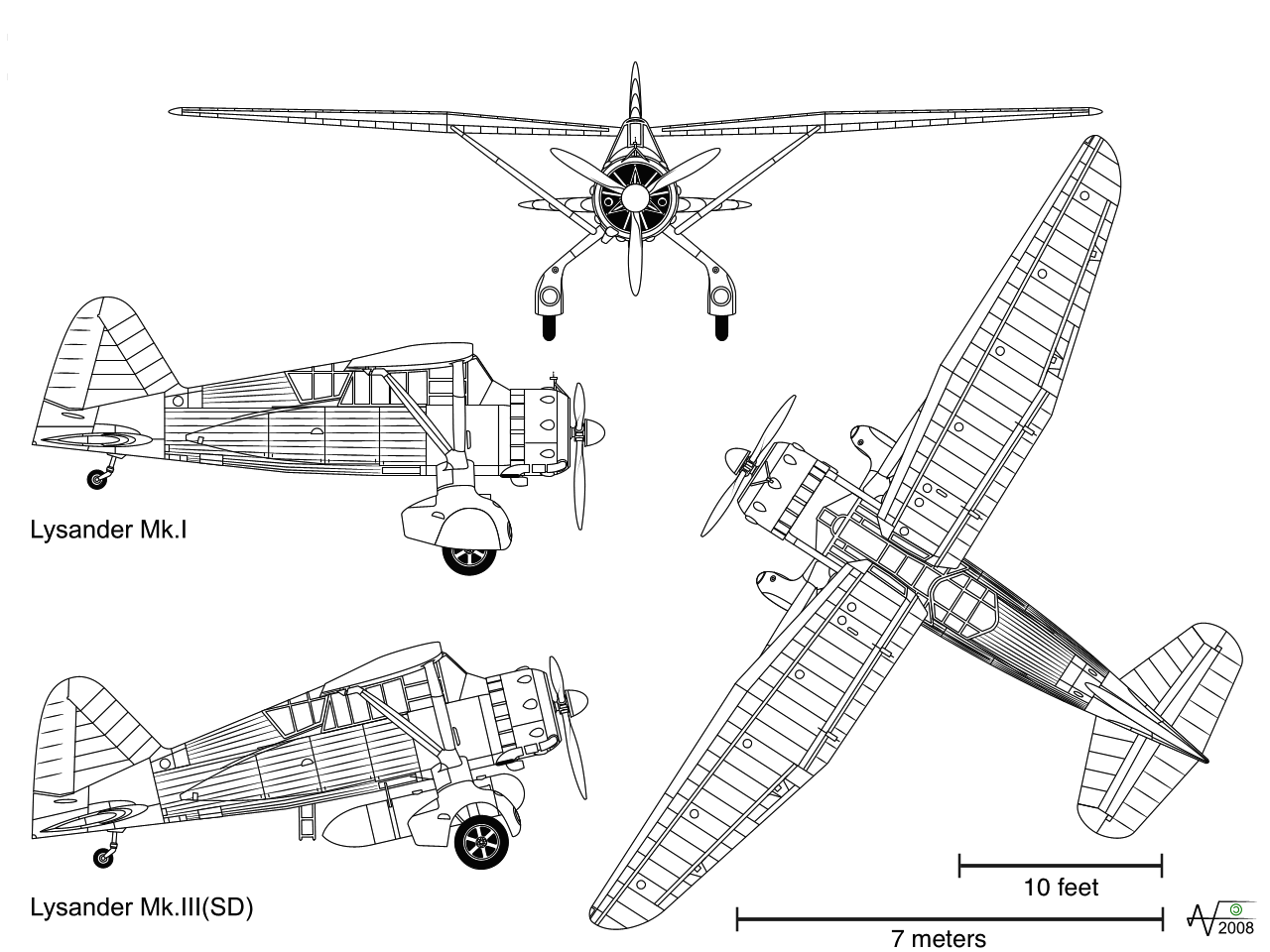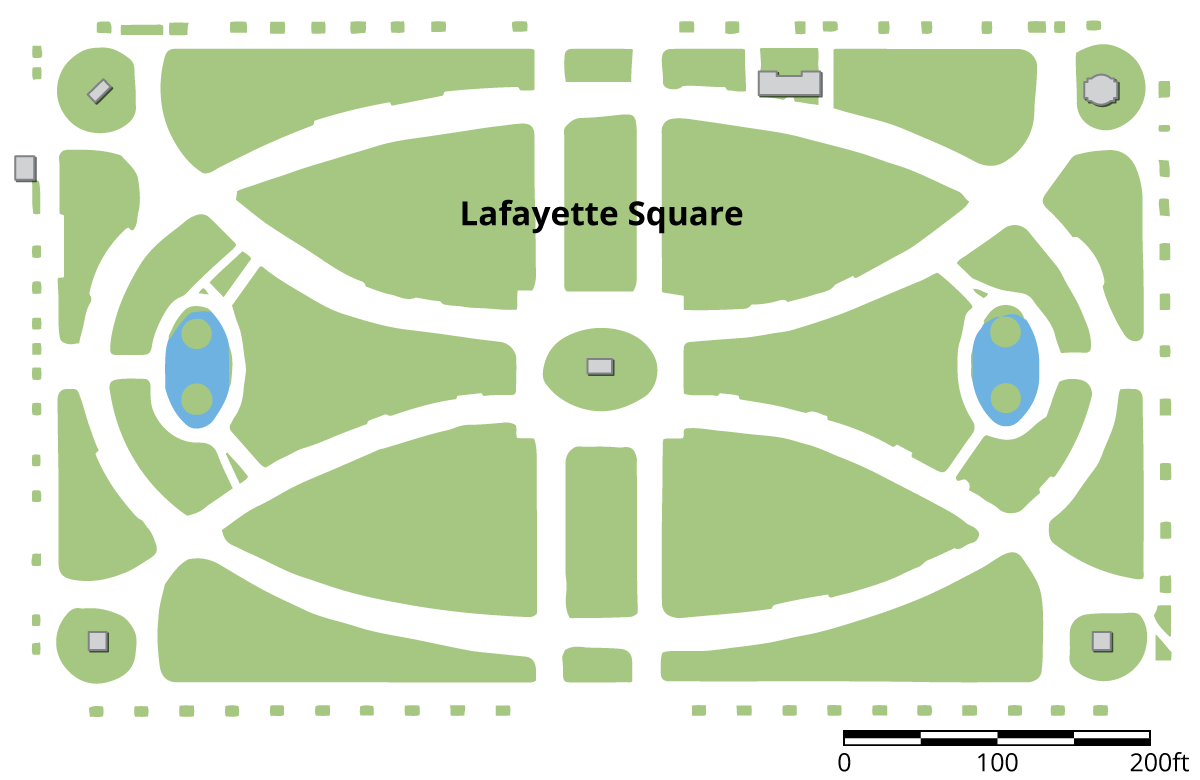PSA6QCK - Practice Problems---7.1 Lesson 7: Scale Drawings (7.G.A.1)
Part A)

The Westland Lysander was an aircraft used by the Royal Air Force in the 1930s. Here are some scale drawings that show the top, side, and front views of the Lysander.Use the scales and scale drawings to approximate the actual lengths of:

the wingspan of the plane, to the nearest foot.

Part B)

The height of the plane, to the nearest foot.

Part C)

The length of the Lysander Mk. I, to the nearest meter.

Part A)

A blueprint for a building includes a rectangular room that measures 3 inches long and 5.5 inches wide. The scale for the blueprint says that 1 inch on the blueprint is equivalent to 10 feet in the actual building. What are the dimensions of this rectangular room in the actual building?

Give the length of the building. Do not include units (feet) in your answer.

Type your answer below as a number (example: 5, 3.1, 4 1/2, or 3/2):
Part B)

Give the width of the building. Do not include units (feet) in your answer.

Type your answer below as a number (example: 5, 3.1, 4 1/2, or 3/2):
Part A)

Here is a scale map of Lafayette Square, a rectangular garden north of the White House.The scale is shown in the lower right corner. Find the actual side lengths of Lafayette Square in feet.

Part B)

Here is a scale map of Lafayette Square, a rectangular garden north of the White House.Use an inch ruler to measure the line segment of the graphic scale. About how many feet does one inch represent on this map?

Part A)

Here is Triangle A. Lin created a scaled copy of Triangle A with an area of 72 square units.How many times larger is the area of the scaled copy compared to that of Triangle A?

Type your answer below as a number (example: 5, 3.1, 4 1/2, or 3/2):
Part B)

What scale factor did Lin apply to the Triangle A to create the copy?

Type your answer below as a number (example: 5, 3.1, 4 1/2, or 3/2):
Part C)

What is the length of bottom side of the scaled copy?